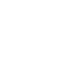An illustrative example of the comparison of calculated and experimental data aimed at increasing the affinity of the ACE2 protein for the Spike protein.
This short presentation allows you to visually compare the results of different methods.The experimental methods are briefly described on the right side of the page, the numerical results are presented in the graph on the left side of the page.The following examples is made without comparison with experimental data
In continuation of the computational work, numerical calculations were performed on the substitutions of amino acid residues in the ACE2 protein
The vectors ACE2 from "0" to "3" are taken from the previous calculation presented in the figure above, the vectors ACE2 from "4" to "7" are described in the table below the graphs.

The last value "7" is in the low range of values lg(cond(W)), so we marked it on the graph with a number, the entropy change (delta)H of the vector "7" is also the maximum.

For the calculation, the obtained 3D model of the biological complex was used by the method of X-ray analysis 7DMU.pdb

The results of numerical calculations allow us to determine the following sequence of ACE2 vectors by increasing affinity for the Spike protein:
Vector "6"
Vector "1"
Vector "4"
Vector "7"